Maths-
General
Easy

Question

# A signal which can be green or red with probability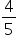and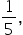respectively, is received by station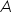and then transmitted to station 8 The probability of each station receiving the signal correctly is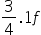the signal received at staTtion8 is green, then the probability that the original signal was green is

## The correct answer is: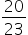### Event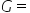original signal is green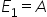receives the signal correct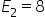receives the signal correct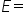signal rreceived by 8 is green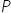(signal received by 8 is green)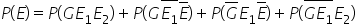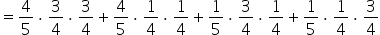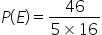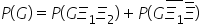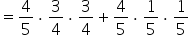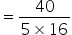Therefore,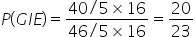Hence, the correct answer is option (c).#### With Turito Foundation.#### Get an Expert Advice From Turito.